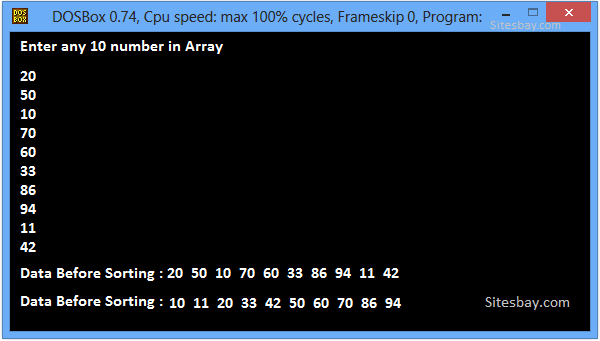# Sort an Array Elements in Ascending Order in C++

## C++ Program to Sort an Array Elements in Ascending Order

Sort an array elements means arrange elements of array in Ascending Order and Descending Order. You can easily sort all elements using bubble sort.
Sort Array Elements in Ascending order means arrange elements of array in Increasing Order.

To understand below Example, you have must knowledge of following C++ programming topics; For Loop in C++ and Swap Program in C++.

### Process of Sorting an Array

The process of sorting an array requires the exchanging of Array values. While this seems to be a simple process, a computer must be careful that no values are lost during this exchange.
Suppose that Marks = 60 and Marks = 80 and you want to exchange their values so that Marks = 80 and Marks = 60. So you could NOT just do this

## Example

```Marks = Marks;
Marks = Marks;  // NOT WORK! Both Marks and Marks Store 80
```

In the first step, the value stored in Marks is erased and replaced with Marks. The result is that both Marks and Marks now have the same value. Oops! Then what happened to the value in Marks? It is lost!!!

In order to swap two values, you must use a third variable, (a "temporary holding variable"), to temporarily hold the value you do not want to lose:

## Example

```temp = Marks;     // holding variable
Marks = Marks;
Marks = temp;
```

This process successfully exchanges, "swaps", the values of the two variables (without the loss of any values).

### Different Ways to Sort Array Elements

There are literally hundreds of different ways to sort arrays. The basic goal of each of these methods is the same: to compare each array element to another array element and swap them if they are in the wrong position.

• Bubble Sort
• Exchange Sort
• Selection Sort
• Insertion Sort
• Shell Sort
• Quick Sort
• Merge Sort

In Below code we sort array Elements in Ascending Order Using Bubble sort (Bubble Sort Program in C++). Bubble sort is based on the idea of repeatedly comparing pairs of adjacent elements and then swapping their positions if they exist in the wrong order

## C++ Program to Sort Elements of Array in Ascending Order

```#include<iostream.h>
#include<conio.h>

void main()
{
int i,a,temp,j;
clrscr();
cout<<"Enter any 10 num in array: \n";
for(i=0;i<=10;i++)
{
cin>>a[i];
}
cout<<"\nData before sorting: ";
for(j=0;j<10;j++)
{
cout<<a[j];
}
for(i=0;i<=10;i++)
{
for(j=0;j<=10-i;j++)
{
if(a[j]>a[j+1])
{
temp=a[j];
a[j]=a[j+1];
a[j+1]=temp;
}
}
}
cout<<"\nData after sorting: ";
for(j=0;j<10;j++)
{
cout<<a[j];
}
getch();
}
```

## Output

```Enter any 10 num in array:
2 5 1 7 5 3 8 9 11 4
Data After Sorting: 1 2 3 4 5 7 8 9 11
```

Output## Pure VPN Privide Lowest Price VPN Just @ \$1.65. Per Month with Non Detected IP Lowest Price Non Detected IP VPN